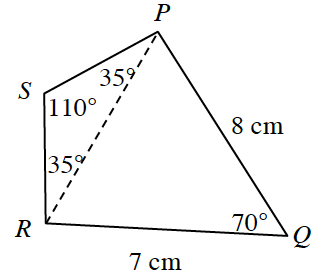### Home > INT3 > Chapter 10 > Lesson 10.2.1 > Problem10-106

10-106.

Use your triangle tools to solve the problems below.1. What is $PR$ in the diagram above?

Use the Law of Cosines to solve for segment $PR$.
Relabel the parts of the triangle so they match the formula if you need more assistance.

Refer to the Math Notes box in Lesson 7.2.3 if you need help remembering what this is.

2. What is the perimeter of quadrilateral $PQRS$?

Use the Law of Sines to find the measure of segment PS.
If you need more assistance refer to Math Note Box in section 7.2.2.

$\frac{\text{sin }35^\circ}{PS}=\frac{\text{sin }110^\circ}{PR}$

Keep in mind $RS = PS$, because $ΔRSP$ is an isosceles triangle, because the base angles are equal at $35^\circ$ each.

Perimeter $= 25.55$ cm